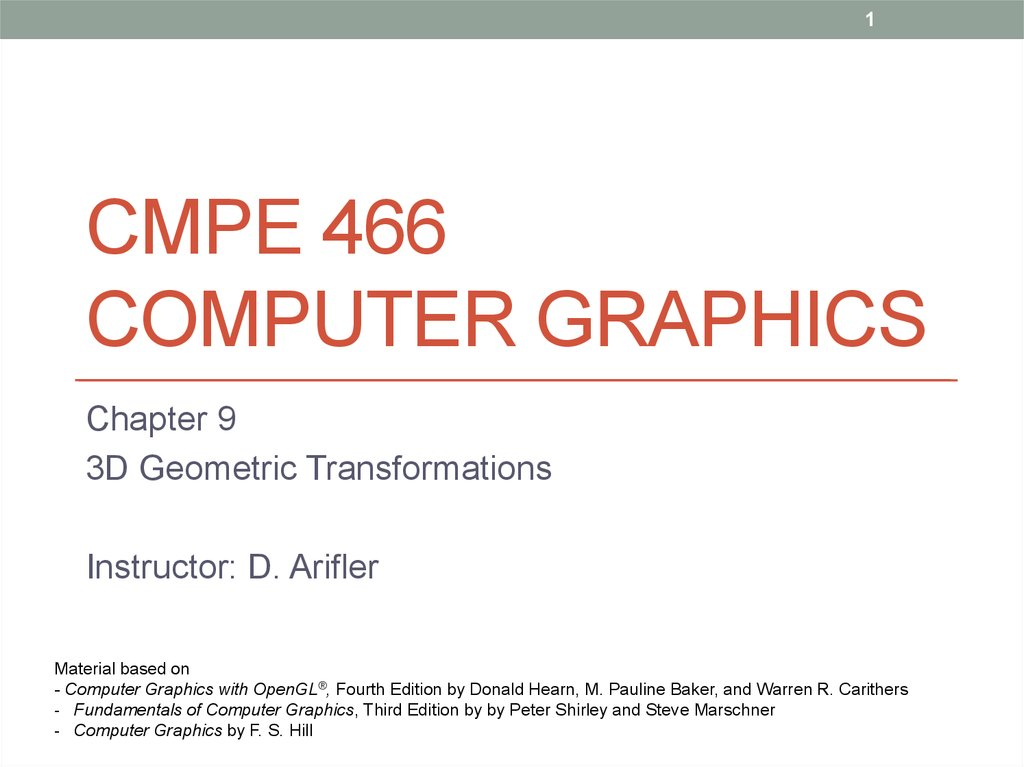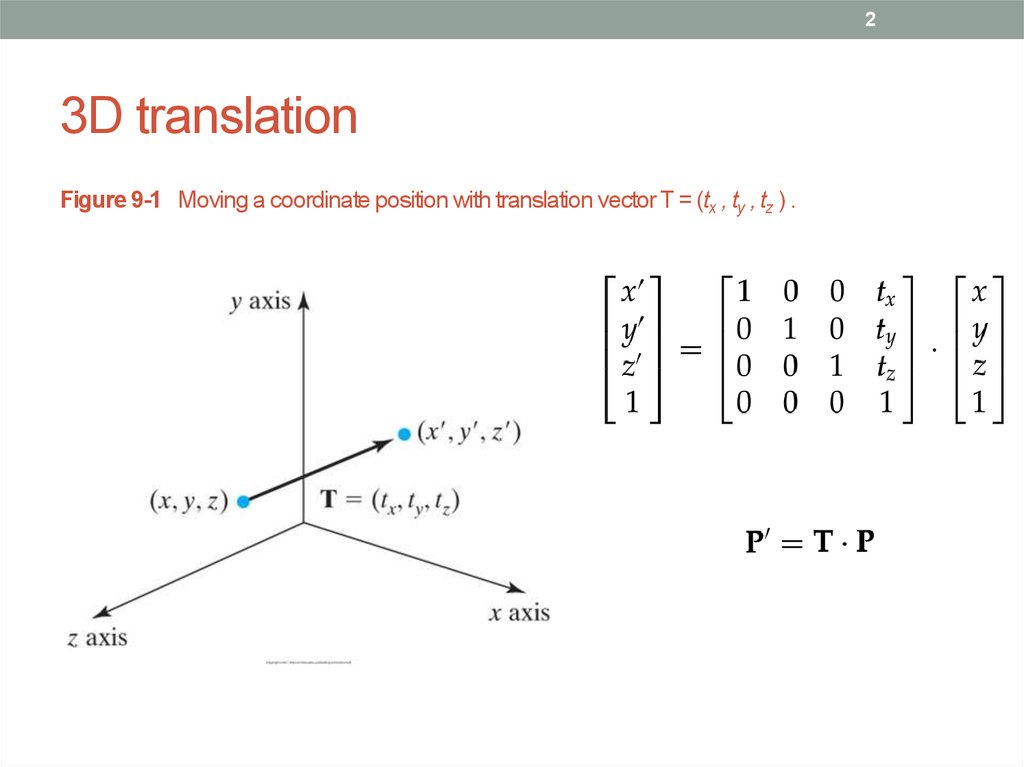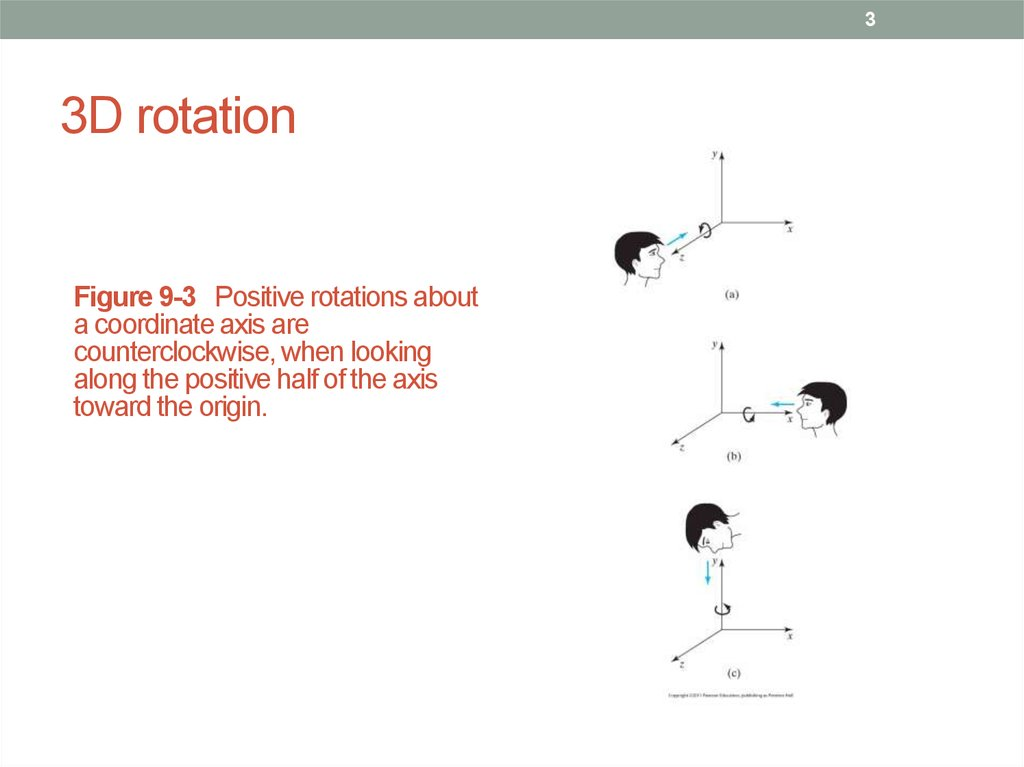# Cmpe 466 computer graphics. 3D geometric transformations. (Сhapter 9)

## 1. CMPE 466 COMPUTER GRAPHICS

1
CMPE 466
COMPUTER GRAPHICS
Chapter 9
3D Geometric Transformations
Instructor: D. Arifler
Material based on
- Computer Graphics with OpenGL®, Fourth Edition by Donald Hearn, M. Pauline Baker, and Warren R. Carithers
- Fundamentals of Computer Graphics, Third Edition by by Peter Shirley and Steve Marschner
- Computer Graphics by F. S. Hill

## 2. 3D translation

2
3D translation
Figure 9-1 Moving a coordinate position with translation vector T = (tx , ty , tz ) .

## 3. 3D rotation

3
3D rotation
a coordinate axis are
counterclockwise, when looking
along the positive half of the axis
toward the origin.

## 4. 3D z-axis rotation

4
3D z-axis rotation
Figure 9-4 Rotation of an object about the z axis.

## 5. Rotations

5
Rotations
• To obtain rotations about other two axes
• x y z x
• E.g. x-axis rotation
• E.g. y-axis rotation

## 6. General 3D rotations

6
General 3D rotations
Figure 9-8 Sequence of transformations for rotating an object about an axis that is
parallel to the x axis.

## 7. Arbitrary rotations

7
Arbitrary rotations
Figure 9-9 Five transformation steps for obtaining a composite matrix for rotation
about an arbitrary axis, with the rotation axis projected onto the z axis.

## 8. Arbitrary rotations

8
Arbitrary rotations
Figure 9-10 An axis of rotation (dashed line) defined with points P1 and P2.
The direction for the unit axis vector u is determined by the specified rotation
direction.

## 9. Rotations

9
Rotations
Figure 9-11 Translation of the rotation axis to the coordinate origin.

## 10. Rotations

10
Rotations
Figure 9-12 Unit vector u is rotated about the x axis to bring it into the xz plane
(a), then it is rotated around the y axis to align it with the z axis (b).

## 11. Rotations

11
Rotations
• Two steps for putting the rotation axis onto the z-axis
Figure 9-13 Rotation of u around the x axis into the xz plane is accomplished by rotating u'
(the projection of u in the yz plane) through angle α onto the z axis.

## 12. Rotations

12
Rotations
• Projection of u in the yz plane
• Cosine of the rotation angle
where
• Similarly, sine of rotation angle can be determined from
the cross-product

## 13. Rotations

13
Rotations
• Equating the right sides
where |u’|=d
• Then,

## 14. Rotations

14
Rotations
• Next, swing the unit vector in the xz plane counter-
clockwise around the y-axis onto the positive z-axis
Figure 9-14 Rotation
of unit vector u'' (vector
u after rotation into the
axis. Positive rotation
angle β aligns u'' with
vector uz .

15
Rotations
and
so that
Therefore

16
Rotations
Together with

## 17. In general

17
In general
Figure 9-15 Local coordinate
system for a rotation axis defined by
unit vector u.

## 18. Quaternions

18
Quaternions
• Scalar part and vector part
• Think of it as a higher-order complex number
• Rotation about any axis passing through the coordinate
origin is accomplished by first setting up a unit quaternion
where u is a unit vector along the selected rotation
axis and θ is the specified rotation angle
• Any point P in quaternion notation is P=(0, p) where p=(x,
y, z)

## 19. Quaternions

19
Quaternions
• The rotation of the point P is carried out with quaternion
operation
where
• This produces P’=(0, p’) where
• Many computer graphics systems use ef cient hardware
implementations of these vector calculations to perform rapid threedimensional object rotations.
• Noting that v=(a, b, c), we obtain the elements for the
composite rotation matrix. We then have

## 20. Quaternions

20
Quaternions
• Using
• With u=(ux, uy, uz), we finally have
• About an arbitrarily placed rotation axis:
• Quaternions require less storage space than 4 × 4
matrices, and it is simpler to write quaternion procedures
for transformation sequences.
• This is particularly important in animations, which often
require complicated motion sequences and motion
interpolations between two given positions of an object.

## 21. 3D scaling

21
3D scaling
Figure 9-17 Doubling the size of an object with
transformation 9-41 also moves the object farther
from the origin.

## 22. 3D scaling

22
3D scaling
Figure 9-18 A sequence of transformations for scaling
an object relative to a selected fixed point, using
Equation 9-41.

## 23. Composite 3D transformation example

23
Composite 3D transformation example

## 24. Transformations between 3D coordinate systems

24
Transformations between 3D coordinate
systems
Figure 9-21 An x'y'z' coordinate system defined within an x y z system. A scene description is
transferred to the new coordinate reference using a transformation sequence that superimposes
the x‘y‘z' frame on the xyz axes.